# Selecting k representative skyline items Representative Skylines using

• Slides: 25Selecting k representative skyline items Representative Skylines using Threshold-based Preference Distributions Atish Das Sarma, Ashwin Lall, Danupon Nanongkai, Richard J. Lipton, Jim Xu College of Computing, Georgia Institute of TechnologySkyline Queries [BKK 01]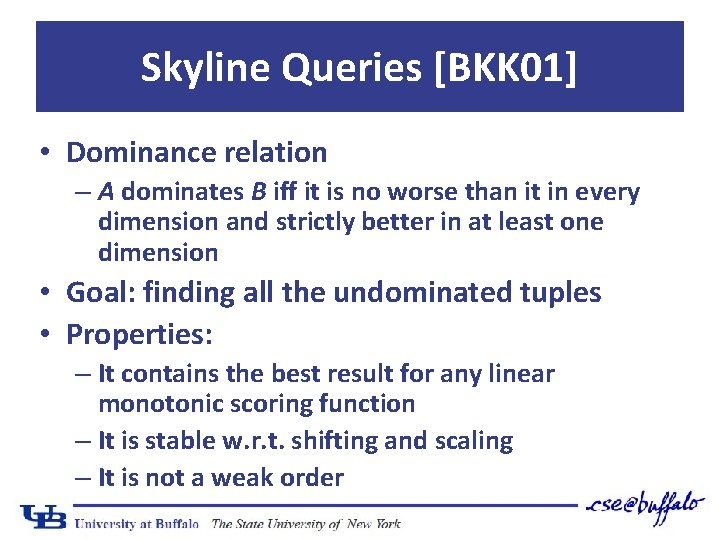Skyline Queries [BKK 01] • Dominance relation – A dominates B iff it is no worse than it in every dimension and strictly better in at least one dimension • Goal: finding all the undominated tuples • Properties: – It contains the best result for any linear monotonic scoring function – It is stable w. r. t. shifting and scaling – It is not a weak order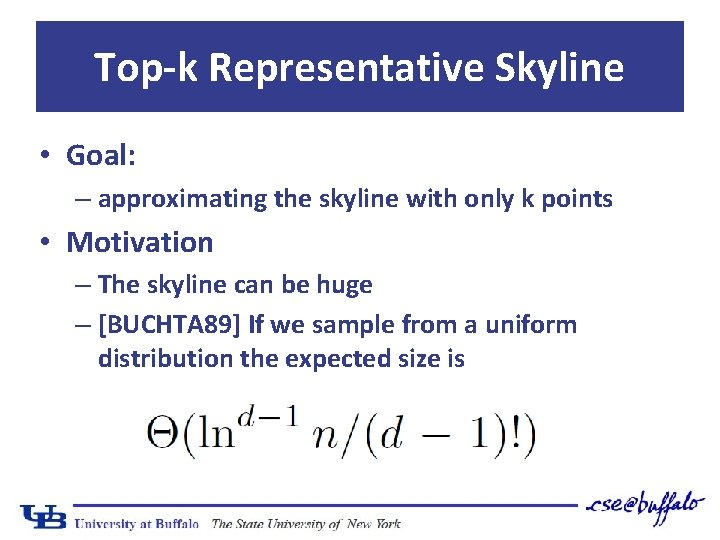Top-k Representative Skyline • Goal: – approximating the skyline with only k points • Motivation – The skyline can be huge – [BUCHTA 89] If we sample from a uniform distribution the expected size isOutline • Three approaches: – Max-dominance [LYZ+07] – Threshold-preference driven [AAD+11] – Distance-based [TDL+09] • Complexity – Two dimensions – Several dimensions • Preference distributions • CritiqueMax-dominance [LYZ+07] • Goal: pick k skyline points such that the total number of data points dominated by at least one of them is maximized • We try to minimize the number of points that are left undominated • Intuition: the user will find interesting those items that dominates many other itemsMax-dominance [LYZ+07]Outline • Three approaches: – Max-dominance [LYZ+07] – Threshold-preference driven [AAD+11] – Distance-based [TDL+09] • Complexity – Two dimensions – Several dimensions • SemanticsThreshold Preferences [AAD+11] • Every user explicitly express her preferences in terms of 0 -1 thresholds • Goal: maximizing the number of users that will click on at least one of the representative points • Note: a skyline point p satisfy a threshold t iff t is dominated by p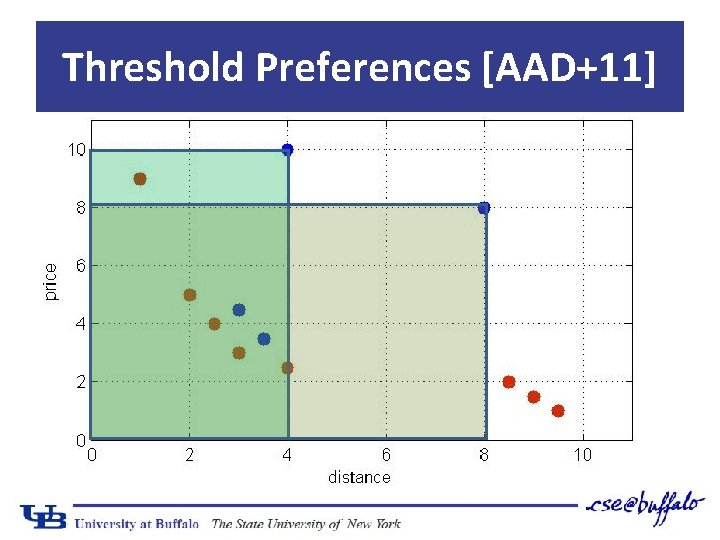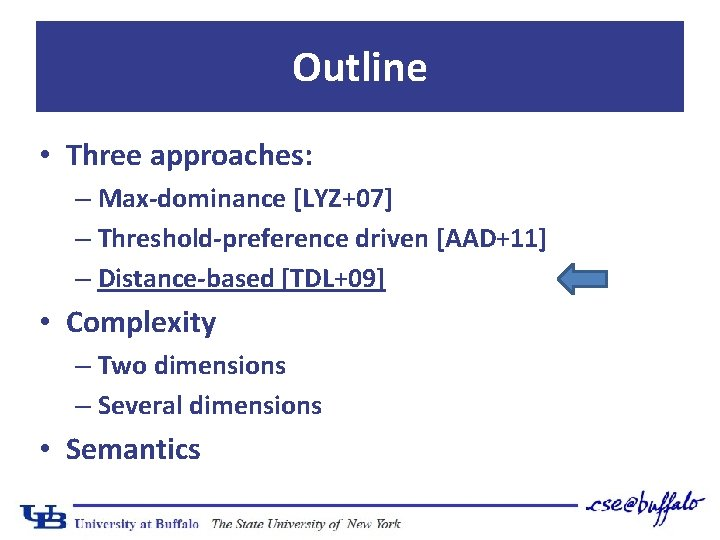Outline • Three approaches: – Max-dominance [LYZ+07] – Threshold-preference driven [AAD+11] – Distance-based [TDL+09] • Complexity – Two dimensions – Several dimensions • Semantics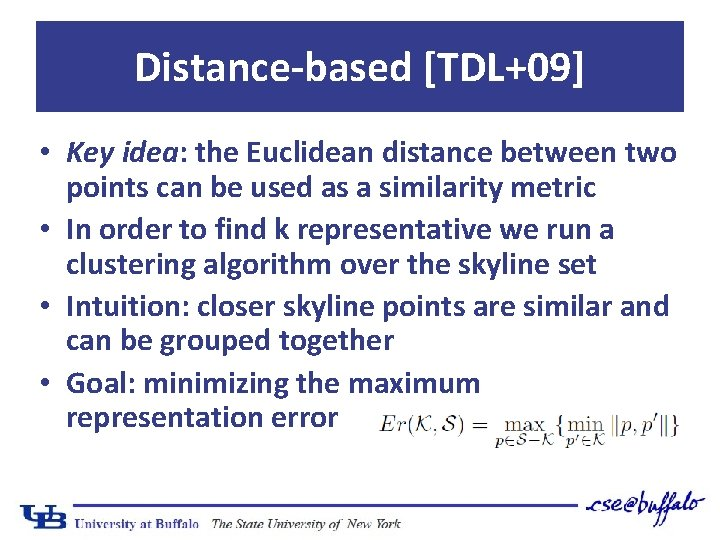Distance-based [TDL+09] • Key idea: the Euclidean distance between two points can be used as a similarity metric • In order to find k representative we run a clustering algorithm over the skyline set • Intuition: closer skyline points are similar and can be grouped together • Goal: minimizing the maximum representation errorDistance-based [TDL+09]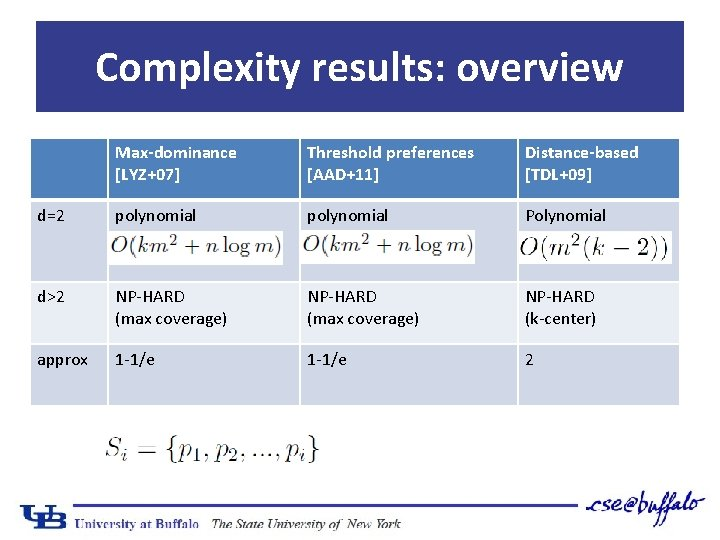Complexity results: overview Max-dominance [LYZ+07] Threshold preferences [AAD+11] Distance-based [TDL+09] d=2 polynomial Polynomial d>2 NP-HARD (max coverage) NP-HARD (k-center) approx 1 -1/e 2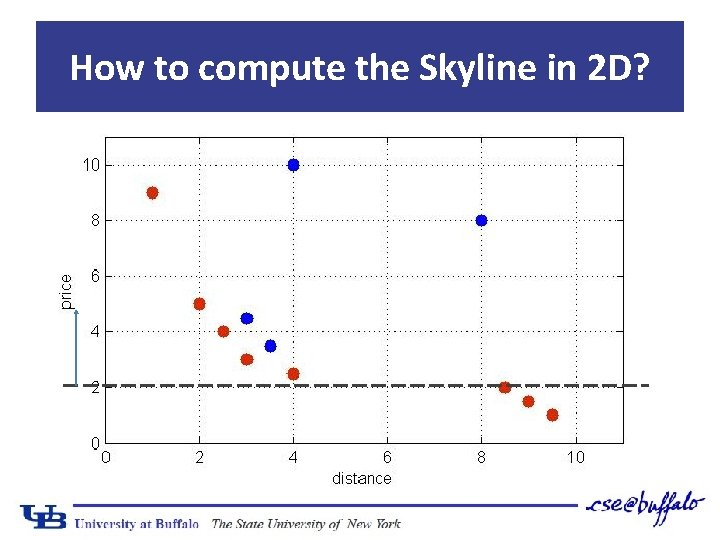How to compute the Skyline in 2 D?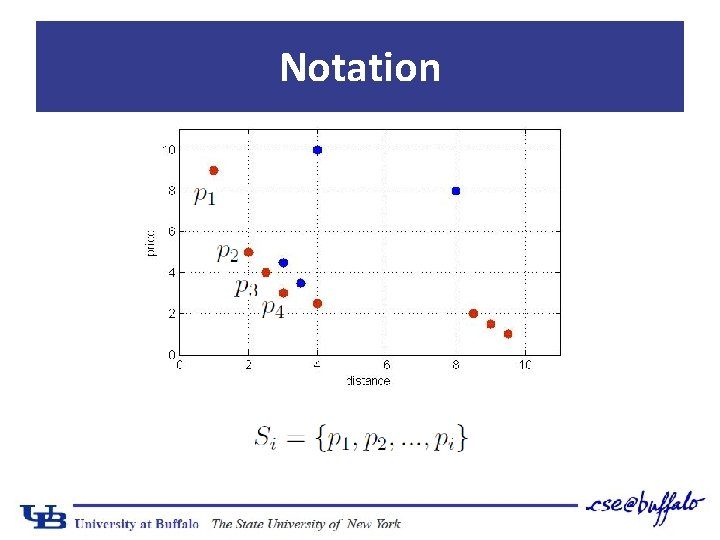Notation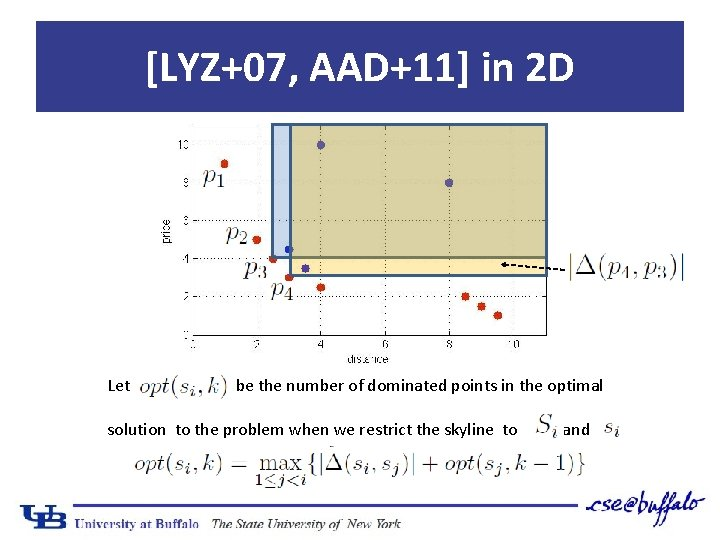[LYZ+07, AAD+11] in 2 D Let be the number of dominated points in the optimal solution to the problem when we restrict the skyline to and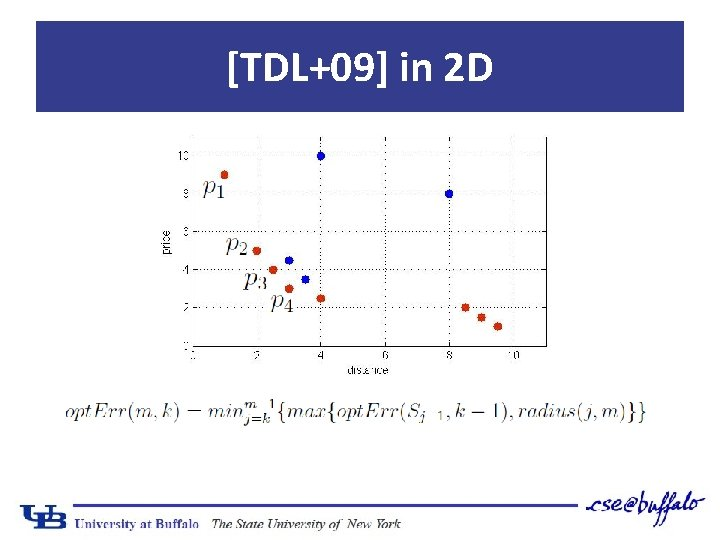[TDL+09] in 2 D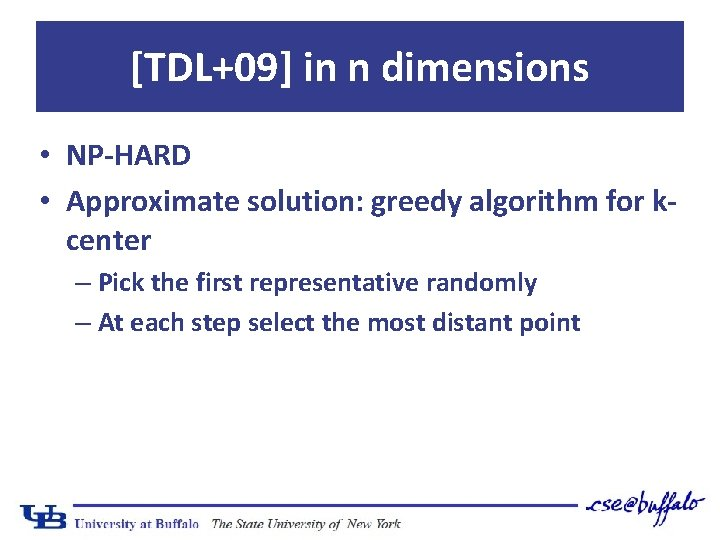[TDL+09] in n dimensions • NP-HARD • Approximate solution: greedy algorithm for kcenter – Pick the first representative randomly – At each step select the most distant point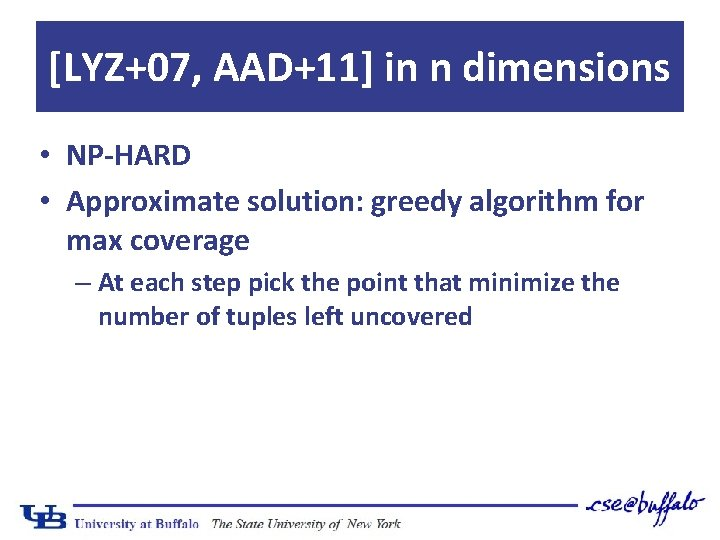[LYZ+07, AAD+11] in n dimensions • NP-HARD • Approximate solution: greedy algorithm for max coverage – At each step pick the point that minimize the number of tuples left uncoveredPreference distributions (F)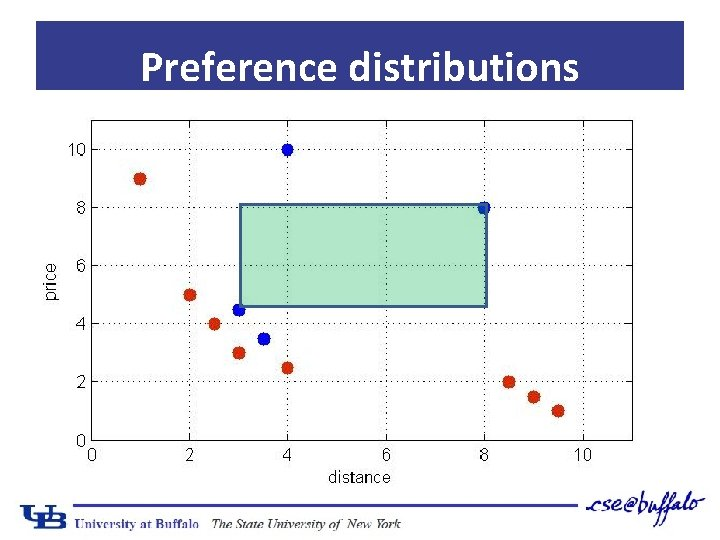Preference distributions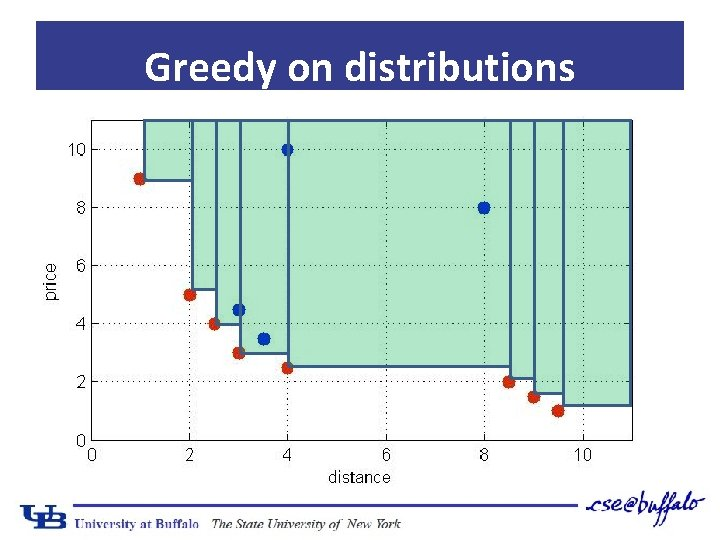Greedy on distributions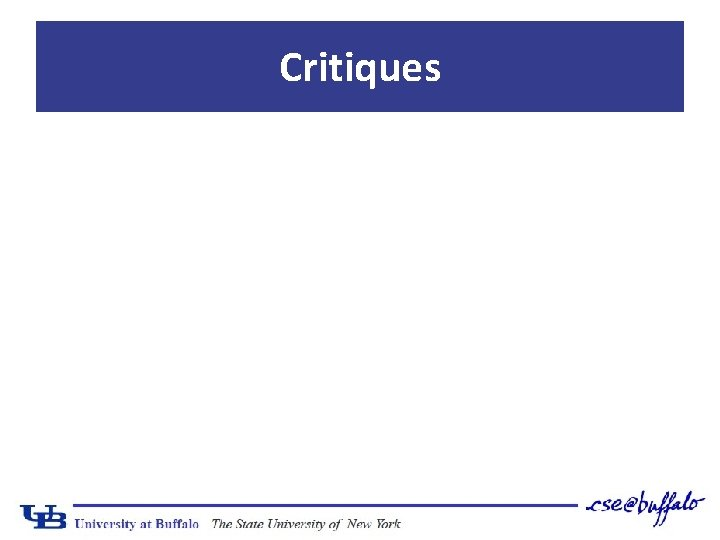Critiques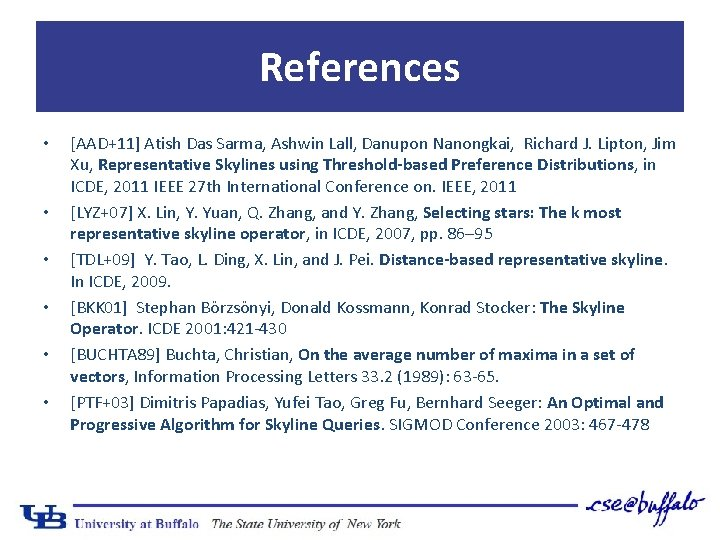References • • • [AAD+11] Atish Das Sarma, Ashwin Lall, Danupon Nanongkai, Richard J. Lipton, Jim Xu, Representative Skylines using Threshold-based Preference Distributions, in ICDE, 2011 IEEE 27 th International Conference on. IEEE, 2011 [LYZ+07] X. Lin, Y. Yuan, Q. Zhang, and Y. Zhang, Selecting stars: The k most representative skyline operator, in ICDE, 2007, pp. 86– 95 [TDL+09] Y. Tao, L. Ding, X. Lin, and J. Pei. Distance-based representative skyline. In ICDE, 2009. [BKK 01] Stephan Börzsönyi, Donald Kossmann, Konrad Stocker: The Skyline Operator. ICDE 2001: 421 -430 [BUCHTA 89] Buchta, Christian, On the average number of maxima in a set of vectors, Information Processing Letters 33. 2 (1989): 63 -65. [PTF+03] Dimitris Papadias, Yufei Tao, Greg Fu, Bernhard Seeger: An Optimal and Progressive Algorithm for Skyline Queries. SIGMOD Conference 2003: 467 -478